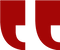### Riemann Zeta

The Riemann zeta function or Euler–Riemann zeta function, ζ(s), is a function of a complex variable s that analytically continues the sum of the infinite series

which converges when the real part of s is greater than 1. More general representations of ζ(s) for all s are given below. The Riemann zeta function plays a pivotal role in analytic number theory and has applications in physics, probability theory, and applied statistics.

This function, as a function of a real argument, was introduced and studied by Leonhard Euler in the first half of the eighteenth century without using complex analysis, which was not available at that time. Bernhard Riemann in his article "On the Number of Primes Less Than a Given Magnitude" published in 1859 extended the Euler definition to a complex variable, proved its meromorphic continuation and functional equation and established a relation between its zeros and the distribution of prime numbers.

The values of the Riemann zeta function at even positive integers were computed by Euler. The first of them, ζ(2), provides a solution to the Basel problem. In 1979 Apéry proved the irrationality of ζ(3). The values at negative integer points, also found by Euler, are rational numbers and play an important role in the theory of modular forms. Many generalizations of the Riemann zeta function, such as Dirichlet series, Dirichlet L-functions and L-functions, are known.A New Math Problem : By @Rafik_Zeraoulia

Is there a fixed integer x >1 satisfing σ^k(x) ≡ 0 (mod x) for all positive integers k ?

Note. σ(x) is the sum of divisors of x, and σ^k(x)=σ(σ(σ(σ(⋯x)))) is σ iterated k times.

Edit. I edited the question to avoid the trivialities.##### Library

To play, press and hold the enter key. To stop, release the enter key.